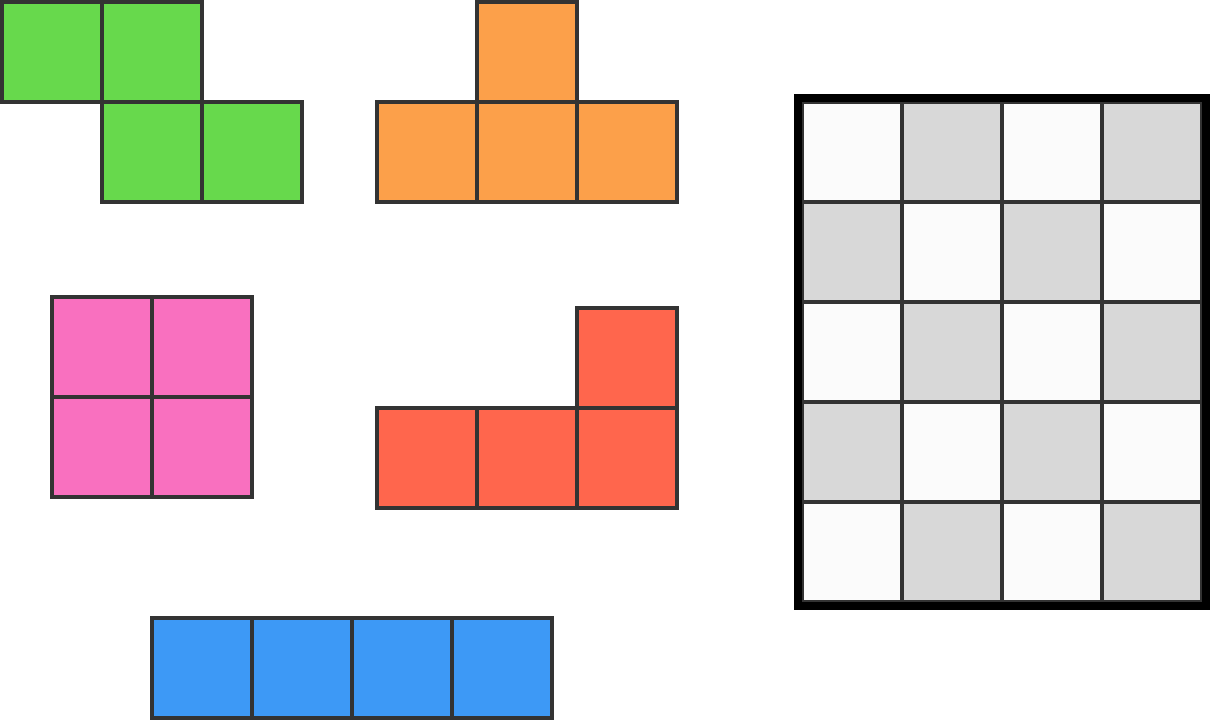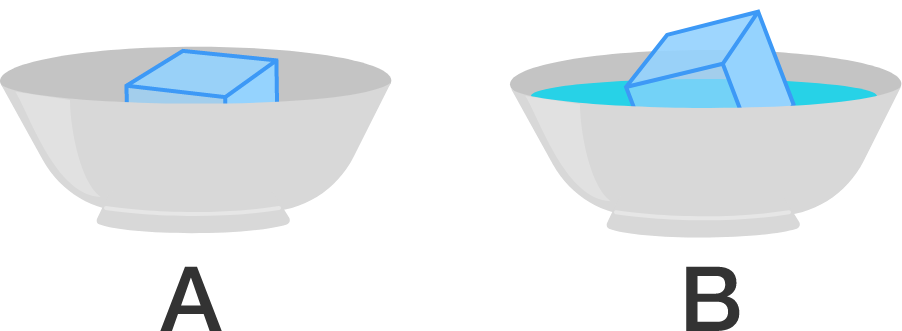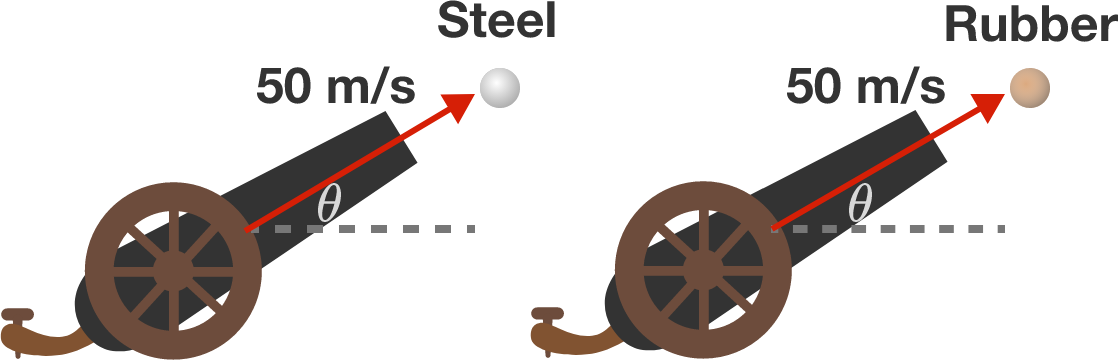# Problems of the Week

Contribute a problem

# 2018-02-26 BasicIf I have the 5 colored shapes shown that I can rotate, and I use each shape once, is it possible to place them so they fit perfectly in a $5 \times 4$ rectangle?

Hint: Notice the checkerboard pattern of the rectangle, and consider how the shapes would be colored if they followed the same pattern.

Suppose you have two identical plastic bowls: bowl A and bowl B. Bowl A is empty, while bowl B is filled with room temperature water. If you place an identical ice cube in each bowl, which bowl's ice cube will melt first?Friends Stella and Robert each have a ball of the same size, except Stella's ball is made of steel, while Robert's is rubber. They are having a competition to see who can launch their ball farthest. If they each have a cannon capable of accelerating their ball to $50\text{ m/s}$ at the same angle of projection, who will win?

Details and Assumptions:

• The balls are shot through the air, but no wind is blowing.
• The distance is measured from the cannon to the point at which the ball first hits the ground.

\begin{aligned} \underbrace{\dfrac23 + \dfrac23 + \cdots + \dfrac23}_{\color{#20A900}{A}\color{#333333} \text{ copies of } \frac23} &= \underbrace{\dfrac45 + \dfrac45 + \cdots + \dfrac45}_{\color{#3D99F6}{B}\color{#333333} \text{ copies of } \frac45} \\\\\\ 20 \leq \color{#20A900}{A}\color{#333333}+\color{#3D99F6}{B}\color{#333333} &\leq 24\\\\ \color{#20A900}{A}\color{#333333}+\color{#3D99F6}{B}\color{#333333} &=\, ? \end{aligned}

I have $\color{#20A900}{A}\color{#333333}$ copies of $\frac23$ on the left-hand side of the equality, and $\color{#3D99F6}{B}\color{#333333}$ copies of $\frac45$ on the right. In total, I've written down between 20 and 24 fractions. Exactly how many fractions are there?

The birthday paradox is a surprising result of probability. Suppose you randomly chose 23 people and put them in a room. Then there would be a good chance $\big($greater than $\frac{1}{2}\big)$ that two of those people share a birthday (even though there are 365 days in the year).

What about birth seasons (spring, summer, fall, winter)? Suppose you randomly chose 3 people and put them in a room. Then is it true that there would be a greater than $\frac{1}{2}$ chance that two of them share a birth season?Note: Birth seasons do not all have the exact same likelihood. However, their likelihoods are close enough that you can assume they are equal for this problem.

×